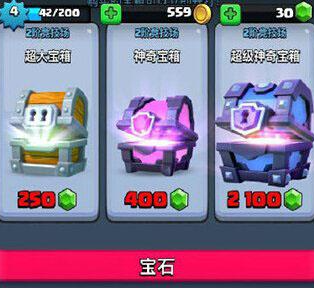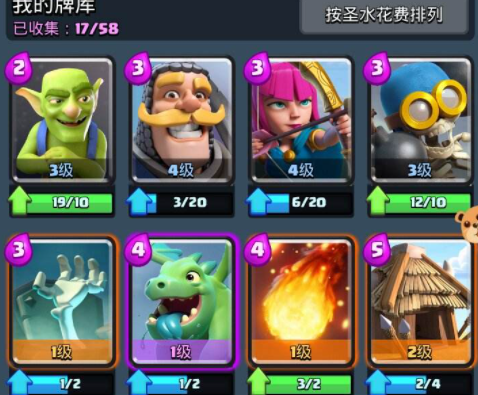# 皇室战争箱子怎么开 教你如何效率开出传说卡

2020年12月23日 14:15 来源：小丑P(一般)+P(稀有)+P(史诗)+P(传说)=1

P(一般)=10*P(稀有)=200*P(史诗)=4000*P(传说)

P(一般)=4000/4421=90.48%

P(稀有)=400/4421=9.048% P(史诗)=20/4421=0.4524% P(传说)=1/4421=0.02262%

1、以超等箱子举例。

A8的超等奇异箱子的信息是：共六百八十四张卡； 最少一百三十六张稀有； 二十二张史诗； 而且有80%几率开出第23张史诗卡。2、以A8的巨人箱子为例，来计算一下其出传奇卡的几率。

A8的巨人箱子的信息是：共三百零四张卡； 最少三十张稀有； 而且有50%几率开出一张史诗卡。

## 游戏推荐### 实名手机号登录注册